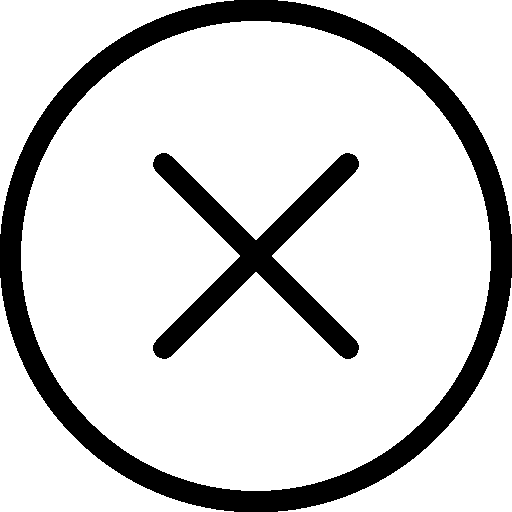• 写文章
• 提问

# 如何实现python字典转字符串?```dict = {'name': '老陈', 'age': 3}
print(type(str(dict)), str(dict))```

1、字典转元组

```print(tuple(dict))
print(tuple(dict.values()))```

2、字典转列表

```tup = (1, 2, 3, 4, 5)
print(tup.__str__())```

3、元组转为列表

`print(list(tup))`